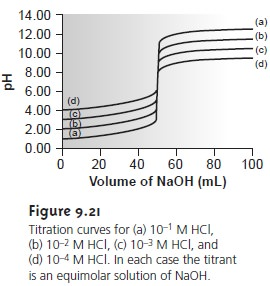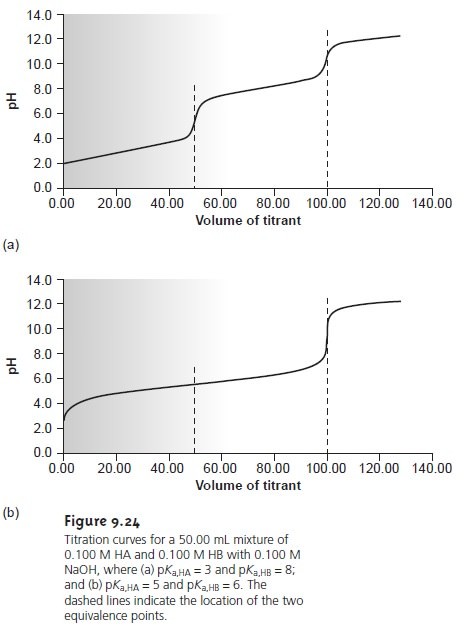Home | | Modern Analytical Chemistry | Evaluation of Acid–Base Titrimetry

# Evaluation of Acid–Base Titrimetry

Scale of Operation: In an acid–base titration the volume of titrant needed to reach the equivalence point is proportional to the absolute amount of analyte present in the analytical solution.

Evaluation of Acid–Base Titrimetry

## Scale of Operation

In an acid–base titration the volume of titrant needed to reach the equivalence point is proportional to the absolute amount of analyte present in the analytical solution. Nevertheless, the change in pH at the equiv- alence point, and thus the utility of an acid–base titration, is a function of the analyte’s concentration in the solution being titrated.When the sample is available as a solution, the smallest concentration of analyte that can be readily analyzed is approximately 10–3 M (Figure 9.21). If, for example, the analyte has a gram formula weight of 120 g/mol, then the lower concentration limit is 120 ppm. When the analyte is a solid, it must first be placed into solution, the volume of which must be sufficient to allow the titration’s end point to be monitored using a visual indicator or a suitable probe. If we assume a minimum volume of 25 mL, and a lower concentration limit of 120 ppm, then a sample containing at least 3 mg of analyte is re- quired. Acid–base titrations involving solid or solution samples, therefore, are generally limited to major and minor analytes. The analysis of gases can be extended to trace analytes by pulling a large vol- ume of the gas through a suitable collection solution.

Efforts have been made to develop methods for conducting acid–base titrations on a much smaller scale. In one experimental design, samples of 20–100 μL were held by capillary action between a flat-surface pH electrode and a stainless steel rod. The titrant was added by using the oscillations of a piezoelectric ceramic device to move an angled glass rod in and out of atube connected to a reservoir containing the titrant (see Figure 9.22). Each time the glass tube was withdrawn an approximately 2-nL microdroplet of titrant was released. The microdroplets were allowed to fall onto the steel rod containing the sample, with mixing accomplished by spinning the rod at 120 rpm. A total of 450 microdroplets, with a combined volume of 0.81–0.84 μL, was dispensed between each pH measurement. In this fashion a titration curve was constructed. This method was used to titrate solutions of 0.1 M HCl and 0.1 M CH3COOH with 0.1 M NaOH. Absolute errors ranged from a minimum of +0.1% to a maximum of –4.1%, with relative standard deviations from 0.15% to 4.7%. The smallest volume of sample that was successfully titrated was 20 μL.More recently, a method has been described in which the acid–base titration is conducted within a single drop of solution.11 The titrant is added using a microbu- ret fashioned from a glass capillary micropipet (Figure 9.23). The microburet has a 1–2 μm tip filled with an agar gel membrane. The tip of the microburet is placed within a drop of the sample solution, which is suspended in heptane, and the titrant is allowed to diffuse into the sample. The titration is followed visually using a col- ored indicator, and the time needed to reach the end point is measured. The rate of the titrant’s diffusion from the microburet must be determined by calibration. Once calibrated, the end point time can be converted to an end point volume. Sam- ples usually consisted of picoliter volumes (10–12 L), with the smallest sample being 0.7 pL. The precision of the titrations was usually about 2%.Titrations conducted with microliter or picoliter sample volumes require a smaller absolute amount of analyte. For example, diffusional titrations have been successfully conducted on as little as 29 femtomoles (10–15 mol) of nitric acid. Nev- ertheless, the analyte must still be present in the sample at a major or minor level for the titration to be performed accurately and precisely.

## Accuracy

When working with macro–major and macro–minor samples, acid–base titrations can be accomplished with relative errors of 0.1–0.2%. The prin- cipal limitation to accuracy is the difference between the end point and the equiva- lence point.

## Precision

The relative precision of an acid–base titration depends primarily on the precision with which the end point volume can be measured and the precision of the end point signal. Under optimum conditions, an acid–base titration can be ac- complished with a relative precision of 0.1–0.2%. The relative precision can be im- proved by using the largest volume buret that is feasible and ensuring that most of its capacity is used to reach the end point. Smaller volume burets are used when the cost of reagents or waste disposal is of concern or when the titration must be com- pleted quickly to avoid competing chemical reactions. Automatic titrators are par- ticularly useful for titrations requiring small volumes of titrant, since the precision with which the volume can be measured is significantly better (typically about 100.05% of the buret’s volume).

The precision of the end point signal depends on the method used to locate the end point and the shape of the titration curve. With a visual indicator, the precision of the end point signal is usually between 0.03 mL and 0.10 mL. End points deter- mined by direct monitoring often can be determined with a greater precision.

## Sensitivity

For an acid–base titration we can write the following general analytical equation

Volume of titrant = k x moles of analyte

where k, the sensitivity, is determined by the stoichiometric relationship between analyte and titrant. Note that this equation assumes that a blank has been analyzed to correct the signal for the volume of titrant reacting with the reagents.

Consider, for example, the determination of sulfurous acid, H2SO3, by titrating with NaOH to the first equivalence point. Using the conservation of protons, we write

Moles NaOH = moles H2SO3There are two ways in which the sensitivity can be increased. The first, and most ob- vious, is to decrease the concentration of the titrant, since it is inversely propor- tional to the sensitivity, k. The second method, which only applies if the analyte is multiprotic, is to titrate to a later equivalence point. When H2SO3 is titrated to the second equivalence point, for instance, equation 9.10 becomesIn practice, however, any improvement in the sensitivity of an acid–base titration due to an increase in k is offset by a decrease in the precision of the equivalence point volume when the buret needs to be refilled. Consequently, standard analytical procedures for acid–base titrimetry are usually written to ensure that titrations re- quire 60–100% of the buret’s volume.

## Selectivity

Acid–base titrants are not selective. A strong base titrant, for example, will neutralize any acid, regardless of strength. Selectivity, therefore, is determined by the relative acid or base strengths of the analyte and the interferent. Two limiting situations must be considered. First, if the analyte is the stronger acid or base, then the titrant will begin reacting with the analyte before reacting with the interferent. The feasibility of the analysis depends on whether the titrant’s reaction with the in- terferent affects the accurate location of the analyte’s equivalence point. If the acid dissociation constants are substantially different, the end point for the analyte can be accurately determined (Figure 9.24a). Conversely, if the acid dissociation con- stants for the analyte and interferent are similar, then an accurate end point for the analyte may not be found (Figure 9.24b). In the latter case, a quantitative analysis for the analyte is not possible.In the second limiting situation the analyte is a weaker acid or base than the in- terferent. In this case the volume of titrant needed to reach the analyte’s equivalence point is determined by the concentration of both the analyte and the interferent. To account for the contribution from the interferent, an equivalence point for the in- terferent must be present. Again, if the acid dissociation constants for the analyte and interferent are significantly different, the analyte’s determination is possible. If, however, the acid dissociation constants are similar, only a single equivalence point is found, and the analyte’s and interferent’s contributions to the equivalence point volume cannot be separated.

## Time, Cost, and Equipment

Acid–base titrations require less time than most gravi- metric procedures, but more time than many instrumental methods of analysis, particularly when analyzing many samples.

With the availability of instruments for performing automated titrations, however, concerns about analysis time are less of a problem. When performing a titration manually the equipment needs are few (a buret and possibly a pH meter), inexpensive, routinely available in most laborato- ries, and easy to maintain. Instrumentation for automatic titrations can be pur- chased for around \$3000.

Study Material, Lecturing Notes, Assignment, Reference, Wiki description explanation, brief detail
Modern Analytical Chemistry: Titrimetric Methods of Analysis : Evaluation of Acid–Base Titrimetry |

Related Topics